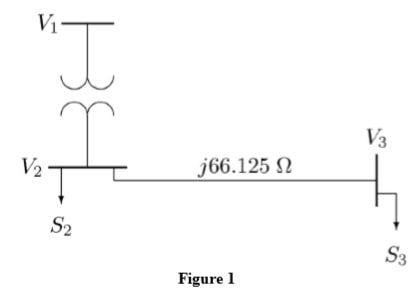# System Analysis ELEN3001 / ELEN6009

```{`Curtin University
Department of Electrical and Computer Engineering Power
System Analysis ELEN3001 / ELEN6009
Assignment 1
`}```

## Assignment PART A

The one-line diagram of a three-phase power system in Figure 1has the following data:

Transformer: 100 MVA, 23/115 kV, X=0.2 Transmission line: 0+j66.125 Ω

Load at bus 2: S2=184.8+j6.6 MVA Load at bus 2: S3=0+j20 MVA

It is required to hold the voltage at bus 3 at 115 kV. Working in per-unit, determine the voltage at buses 2 and 1. Use a base of 100 MVA and neglect the transformer resistance, and phase shiftFigure 1

## Assignment PART B

B.1) Show that the generalized circuit constants of all three transmission-line models (short, medium and long) satisfy the condition below:

B.2) A 60 Hz three-phase transmission line is 300 mi long and serves a load of 400 MVA, with 0.8 lagging power factor at 345 kV. The ABCD constants of the line are:

A= D= 0.818∠1.3°

B=172.2∠84.2° Ω

C = 0.001933∠90.4° S

1. Determine the sending-end line-to-neutral voltage, the sending-end current and the sending-end active power.
2. Percent voltage regulation
3. Transmission line efficiency at full load
4. Determine the line propagation constant γ and the Characteristic impedance Zc
5. Calculate the total series impedance and total shunt admittance of the line
6. Draw the equivalent π-circuit and calculate its parameters (Z \ and Y \ ) 2
7. Calculate the wavelength λ and velocity of propagation
8. If the line is lossless (i.e. R=G=0); Calculate the surge impedance
9. If the line is loaded with the surge impedance; Calculate the SIL
10. Re-calculate the ABCD parameters for the lossless TL
11. Re-calculate the line efficiency and voltage regulation for the lossless line.